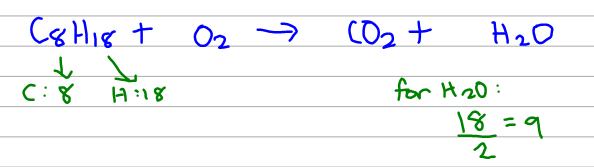# Problem: The combustion of gasoline produces carbon dioxide and water. Assume gasoline to be pure octane (C8H18) and calculate the mass (in kg) of carbon dioxide that is added to the atmosphere per 2.1 kg of octane burned. (Hint: Begin by writing a balanced equation for the combustion reaction.)

###### FREE Expert Solution

First, write the reaction without the coefficients with octane (C8 H18 ) and oxygen (O2) on the reactant side and carbon dioxide (CO2) and water (H2O) on the product side. These products are standard for the combustion of a hydrocarbon.94% (260 ratings)###### Problem Details

The combustion of gasoline produces carbon dioxide and water. Assume gasoline to be pure octane (C8H18) and calculate the mass (in kg) of carbon dioxide that is added to the atmosphere per 2.1 kg of octane burned. (Hint: Begin by writing a balanced equation for the combustion reaction.)